# Circular Motion & Rotational Dynamics

#### 13. Angular Momentum

13. Angular Momentum
(a) The moment of linear momentum of a body with respect to any axis of rotation is known as angular momentum.
(b) It is a vector quantity, which is often represented byor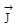(c) Angular momentum=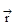×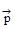=x (m)
= m (x)
or= rp sin θ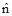= mvr sin θθ is angle betweenand,is unit vector perpendicular to the plane ofand.
(d) The direction of angular momentum is perpendicular to the plane ofandand it is given by right hand screw rule.
(e) J = mvr sin θ
Cases :
(I) If θ = 0, J = 0 ¼ Minimum ½
(II) If θ = 90°, J = mvr ¼Maximum½ = (mr2) ω : Q v = rω.
(f) Unit : J. second , kg m2/s, kg m2 rad/sec
(g)dimension : [M1L2T–1]
(h) If direction of rotation is anticlockwise, angular momentum is taken positive and if direction of rotation is clockwise, angular momentum is taken negative.
(i) The angular momentum of a system of particle's is equal to the vector sum of angular momentum of each particle.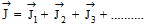(j) Relation between angular momentum and angular velocity
J = I ω
I - Moment of inertia with respect to axis of rotation
ω - Angular velocity due to angular momentum
J - The moment of momentum which is causing rotational motion.
(k) The rate of change of angular momentum is equal to the torque applied on the body.(l) In rotational motion angular momentum has equal importance as linear momentum in linear motion
(m) If torque acting of a particle is zero then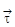= 0 ⇒= 0
Which implies that the angular momentum remains conserved when no external force acts on the body.
13.1 Law of Conservation of Angular Momentum
(a) If no external torque is acting upon a body rotating about an axis, then the angular momentum of the body remains constant that is, J = Iω = constant
(b) Proof : We have read above that when a body rotates about an axis under the action of an external torque t, the rate of change of angular momentum of the body is equal to the torque; that is,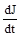= τ
If the external torque is zero (τ = 0),= 0 ⇒ J = constant
This is the law of conservation of angular momentum.
Examples :-(i) Suppose a ball is tied at one end of a cord whose other end passes through a vertical hollow tube. The tube is held in one hand and the cord in the other. The ball is set into rotation in a horizontal circle. If the cord is pulled down, shortening the radius of the circular path of the ball, the ball rotates faster than before. The reason is that by shortening the radius of the circle, the moment of inertia of the ball about the axis of rotation decreases. Hence, by the law of conservation of angular momentum, the angular velocity of the ball about the axis of rotation increases. [fig. (1)]
ii) When a diver jumps into water from a height, he does not keep his body straight but pulls in his arms and legs towards the centre of his body. On doing so, the moment of inertia I of his body deceases. But since the angular momentum I ω remains constant, his angular velocity ω correspondingly increases. Hence during jumping he can rotate his body in the air. [fig. (2)]
iii) In a man with his arms outstretched and holding heavy dumb bells in each hand, is standing at the centre of a rotating table. When the man pulls in his arms, the speed of rotation of the table increases. The reason is that on pulling in the arms, the distance R of the dumbbells from the axis of rotation decreases and so the moment of inertia o the man decreases. Therefore, by conservation of angular momentum, the angular velocity increases. [fig. (3)]
In the same way, the ice skater and the ballet dancer increase or decrease the angular velocity of spin about a vertical axis by pulling or extending out their limbs.

If you want to give information about online courses to other students, then share it with more and more on Facebook, Twitter, Google Plus. The more the shares will be, the more students will benefit. The share buttons are given below for your convenience.

#### IIT (Class X)

• Unit, Dimension & Error
• Vectors
• Motion in One Dimension
• PROJECTILE MOTION
• NEWTON'S LAWS OF MOTION & FRICTION
• WORK, POWER, ENERGY & CONSERVATION LAWS
• CIRCULAR MOTION & ROTATIONAL DYNAMICS
• GRAVITATION